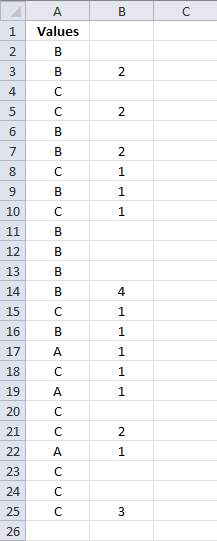Author: Oscar Cronquist Article last updated on April 23, 2017

Hi Oscar,
In column A, I have a long random list of two variables, "N/A" and the value 1. In column B I want to identify the number of contiguous occurrences of the value 1 before the next appearance of "N/A". I wonder if you could point me to the best way of achieving this please? Example below shows what I am after.Much appreciation for your excellent website,
DavidCol A Col B
N/A
N/A
1
1
1
1
1 5
N/A
1
1
1
1 4
N/A
N/A
1
1 2
N/A
N/A
N/A
1 1

Thanks, David.

The following formula looks for contiguous values, not only 1 and N/A.Array formula in cell B3:

=IF(SUM(IF(\$A\$2:A3<>\$A\$3:A4, 1, 0))=SUM(IF(\$A\$2:A2<>\$A\$3:A3, 1, 0)), "", INDEX(FREQUENCY(IF(\$A\$2:\$A\$25<>\$A\$3:\$A\$26, "", MATCH(ROW(\$A\$2:\$A\$25), ROW(\$A\$2:\$A\$25))), IF(\$A\$2:\$A\$25<>\$A\$3:\$A\$25, MATCH(ROW(\$A\$2:\$A\$25), ROW(\$A\$2:\$A\$25)), ""))+1, SUM(IF(\$A\$2:A3<>\$A\$3:A4, 1, 0))))

How to enter an array formula

1. Select cell B3
2. Paste above formula to formula bar
3. Press and hold CTRL + SHIFT simultaneously
4. Press Enter once
5. Release all keys

Copy cell B3 and paste to cells below as far as needed.